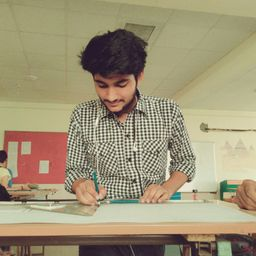Ask your homework questions to teachers and professors, meet other students, and be entered to win \$600 or an Xbox Series X 🎉Join our Discord!Numerade Educator

# A 0.110-nm photon collides with a stationary electron. After the collision, the electron moves forward and the photon recoils backwards. Find (a) the momentum and (b) the kinetic energy of the electron.

## no answer available

Quantum Physics

Atomic Physics

### Discussion

You must be signed in to discuss.
##### Top Physics 103 Educators##### Christina K.

Rutgers, The State University of New Jersey

LB##### Jared E.

University of Winnipeg### Video Transcript

here for the solution Wavelength Lambda for Tony 0.11 and M, which is equal to 0.11 multiplied by 10 to the power minus 9 m. Frank Constant is magical to 6.63 Multiply by 10 to the power my 34 j s mass. That is M see off a me off electron that 9.11 multiplied by 10 to the power minus 31 kg. Charge E on electron is 1.6, multiplied by 10 to the power minus nine. See scattering angle. Tita is equal to 1 80 degree now if Lambda equal to act by m Easy, then we can write the shift Delta Lambda is given by the Delta Lambda is equal to Lambda See Bracket one minus cost 1 80 degree hair by soldiers. We get 0.486 and m. Now the is scattered wavelength that is Lambda tm will be Lambda is equal to Lambda Plus Delta Lambda is equal to 0.110 plus 0.486 and which is equal to 0.115 n. M. The kinetic energy that s k off the recall electron will be That s K is equal to FC Lambda Delta Lambda Divide by Lambda Lambda Days. Now here we substituted the values off. Everybody will that etc Delta, Lambda, Lambda and Lambda Desk. And by calculating this, we get 478 Evie. So now the Mo mentum p off the recoil electron will be p equal to on the road. Um, e k g Here we substituted the values and sold for this. We got 1.18 multiply by 10 to the power minus 23 kg meter per second. And this is our final answer. So this is a complete solution. The step by step in until please go through this.DR APJ ABDUL KALAM TECHNICAL UNIVERSITY

#### Topics

Quantum Physics

Atomic Physics

##### Top Physics 103 Educators##### Christina K.

Rutgers, The State University of New Jersey

LB##### Jared E.

University of Winnipeg